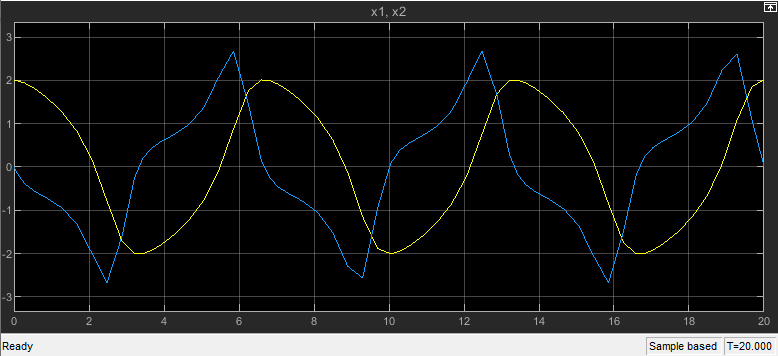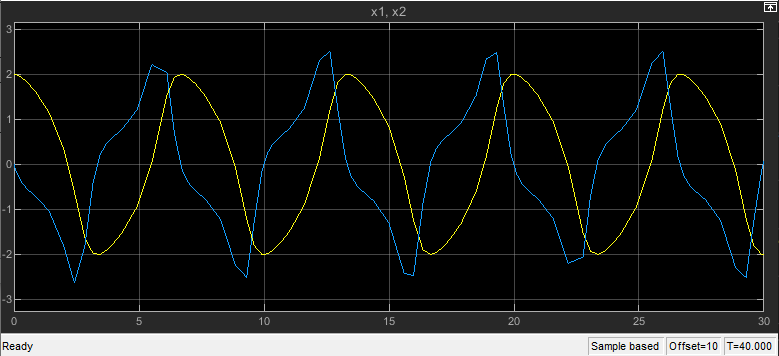# Start time

Simulation start time

Model Configuration Pane: Solver

## Description

Specify the start time for the simulation in seconds, as a double-precision value. The start time specifies the first time value for which the simulation computes a result and the time from which the simulation engine propagates time.

## Settings

`0.0` (default) | scalar number
• The start time must be less than or equal to the stop time.

• The values of block parameters with initial conditions must match the initial condition settings at the specified start time.

• Simulation time is not the same as clock time. For example, running a simulation for 10 seconds usually does not take 10 seconds. Total simulation time depends on many factors, such as model complexity, solver step size, and system speed.

• When you use a fixed-step solver, the start time for the simulation must be zero or an integer multiple of the fixed time step for the simulation. When you specify a start time that does not satisfy this requirement, the software issues a diagnostic and changes the start time to the nearest integer multiple of the fixed step size. To change the diagnostic behavior for this condition, use the Automatic solver parameter selection parameter.

## Examples

expand all

Open the model `vdp`.

```mdl = "vdp"; open_system(mdl)```

The model is saved with a start time of `0` seconds and a stop time of `20` seconds.

`get_param(mdl,"StartTime")`
```ans = '0.0' ```
`get_param(mdl,"StopTime")`
```ans = '20' ```

Simulate the model. To view the simulation results, double-click the Scope block. The Scope window displays the results from the start time to the stop time.

`out1 = sim(mdl);`Change the start time to `10` seconds and the stop time to `40` seconds.

1. On the Modeling tab, under Setup, click Model Settings.

2. Select the Solver pane.

3. In the Start time box, enter `10`.

4. In the Stop time box, enter `40`.

5. Click OK.

Alternatively, use the `set_param` function to configure the start and stop time programmatically.

`set_param(mdl,"StartTime","10","StopTime","40")`

Simulate the model again. The Scope window updates to reflect the longer simulation time. The time axis ranges from 0 to 30, with a 10-second offset indicated in the lower-right of the Scope window.

`out2 = sim(mdl);`To change the start and stop time for a simulation without modifying configuration parameter values saved in a model, use a `Simulink.SimulationInput` object.

Open the model `vdp`.

```mdl = "vdp"; open_system(mdl)```

As saved, the model has a start time of `0` seconds and a start time of `20` seconds.

`get_param(mdl,"StartTime")`
```ans = '0.0' ```
`get_param(mdl,"StopTime")`
```ans = '20' ```

Create a `Simulink.SimulationInput` object to configure a simulation of the model.

`simIn = Simulink.SimulationInput(mdl);`

Use the `setModelParameter` function to specify a start time of `10` seconds and a stop time of `40` seconds for the simulation.

```simIn = setModelParameter(simIn,"StartTime","10",... "StopTime","40");```

Simulate the model using the `SimulationInput` object.

`out = sim(simIn);`

The simulation uses the start time and stop time values defined on the `SimulationInput` object.

`tFirst = out.yout{1}.Values.Time(1)`
```tFirst = 10 ```
`tLast = out.yout{1}.Values.Time(end)`
```tLast = 40 ```

The configuration parameter values in the model remain unchanged.

`get_param(mdl,"StartTime")`
```ans = '0.0' ```
`get_param(mdl,"StopTime")`
```ans = '20' ```

## Recommended Settings

The table summarizes recommended values for this parameter based on considerations related to code generation.

ApplicationSetting
DebuggingNo impact
TraceabilityNo impact
EfficiencyNo impact
Safety precaution`0.0`

## Programmatic Use

 Parameter: `StartTime` Type: string | character vector Values: scalar double Default: `'0.0'`

## Version History

Introduced before R2006a